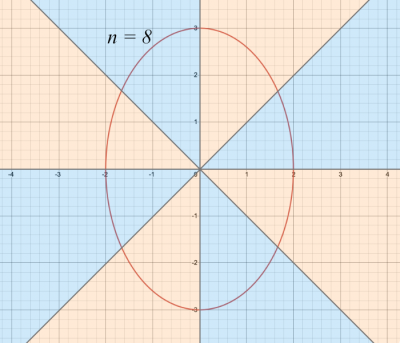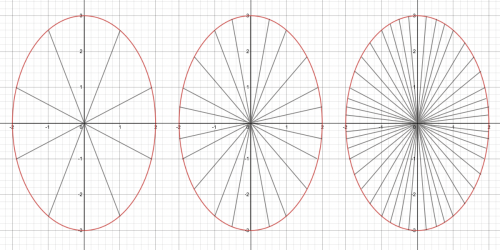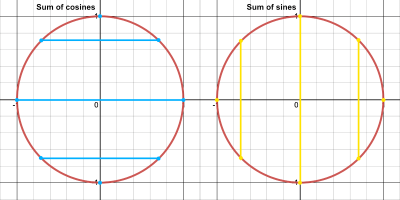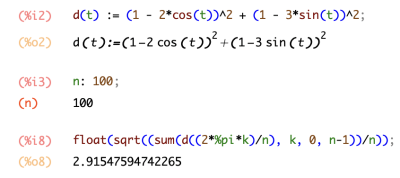I'm a full stack software engineer with a particular interest in functional programming and mathematics in general. Here you can find a history of my professional roles as well as my own side projects and articles.

# Finding the average distance from an ellipse to a point

18th Dec 2021

## Motivation

I've recently been reading a book called Infinite Powers by Steven Strogatz about the history that led to the development of calculus. This, combined with the subject of conics which I've been studying as part of my university work, led me to start thinking about the Astronomical Unit and how it's calculated.

## A Problem

My understanding of the Astronomical Unit was that it represents the average distance between the Earth and the Sun. This understanding leads to an interesting problem: the Earth orbits around the Sun in an ellipse, and there are infinitely many points on that ellipse at which you could measure the distance between the Earth and the Sun. How can we take an average of infinitely many things? This sounds like a problem well suited to calculus.

As it turns out, the Astronomical Unit was originally calculated by taking the average of the Earth's aphelion and perihelion (the furthest and closest points to the sun, respectively).

\begin{align*} \text{Astronomical Unit} = \frac{\text{Aphelion} + \text{Perihelion}}{2} \end{align*}

So it's quite a rough average. I therefore set myself the challenge of finding the true average - the average of the infinitely many distances from the sun to points along the Earth's orbit.

In this post, I'll outline the process I went through to find a general formula for finding the true average of the distances from an arbitrary point to all the possible points along an ellipse. I'll save the final step of deriving the equation of the Earth's elliptical orbit and applying the formula to it for another post.

## The Strategy

I'll start this section by saying that I'm an undergraduate student just doing this for fun, so do take my conclusions with some skepticism.

I reasoned that if we can find a function $f(\theta)$ for the distance from an arbitrary point $(p,q)$ to the point on the ellipse which coincides with the straight line extending from the origin at angle $\theta$, we could then take $n$ evenly spaced values of $\theta$ and take the average of their respective $f(\theta)$ values.

\begin{align*} \text{Rough Average Distance} = \frac{\sum_{k=0}^{n - 1} f\left(\frac{2\pi \times k}{n}\right)}{n} \end{align*}

Notice that $\theta$ is replaced with $\frac{2\pi \times k}{n}$. Dividing $2\pi$ by $n$ divides the circle around the origin into the appropriate number of slices, and then we multiply the result by $k$ to select the appropriate slice. $k$ effectively becomes an index.$2\pi$ divided into $n = 8$ slices

It's important for the values of $\theta$ to be evenly spaced so as not to introduce a bias towards one or another extreme of the ellipse. The fact that the angles are evenly spaced also makes simplifying the equation much simpler later on, as we'll see.

This average would increase in precision as you take more and more distances (as $n$ increases) - much like how a mean average better represents the population as the sample size increases. Continuing the statistics analogy, the population we're dealing with is infinitely large, meaning no matter how large (but finite) our sample size $n$, the result will still only be a rough average.Average gets more accurate as $n\to\infty$

The true average distance would be the limit as $n$ approaches infinity.

\begin{align*} \text{True Average Distance} = \lim_{n\to\infty} \frac{\sum_{k=0}^{n - 1} f\left(\frac{2\pi \times k}{n}\right)}{n} \end{align*}

So the strategy can be summarised as follows:

1. Find a function for the distance from a given point on an ellipse in standard position to an arbitrary point $(p,q)$.
2. Find a function for the average of the distances between $n$ evenly spaced points on the ellipse and the point $(p,q)$ (using the function found in part 1).
3. Find the limit of the function found in part 2 as $n \to \infty$.

## Step 1: A function for the distance from $(p,q)$ to a point on the ellipse

To find a function for the distance from a point on the ellipse to the point $(p,q)$, we can start with the standard formula for the distance between two points

\begin{align*} \text{Distance} = \sqrt{(x_2 - x_1)^2 + (y_2 - y_1)^2} \end{align*}

but since we're looking for a function, we'll need to square both sides. This will just mean taking the square root as a final step in calculating the average. So the function will take the form

\begin{align*} d(x) = (p - x)^2 + (q - y)^2 \end{align*}

where $x$ and $y$ are the coordinates of the point on the ellipse.

The equation of any ellipse in standard position takes the form

\begin{align*} \frac{x^2}{a^2} + \frac{y^2}{b^2} = 1 \end{align*}

where $a$ and $b$ are constants. However, this can be parametrised into two separate formulas for $x$ and $y$.

\begin{align*} x &= a\cos{\theta} \\ y &= b\sin{\theta} \end{align*}

Therefore, our function for the square of the distance will be

\begin{align*} d(\theta) = (p - a\cos{\theta})^2 + (q - b\sin{\theta})^2 \end{align*}

## Step 2: A function for the average of $n$ distances

Now that we have a function $d(\theta)$ for a single square distance from $(p,q)$ to a point on the ellipse, the function $D(n)$ for the average of $n$ distances will take the form

\begin{align*} D(n) = \frac{\sum_{k = 0}^{n - 1} d\left(\frac{2\pi k}{n}\right)}{n} \end{align*}

For small values of $n$, this is quite simple to calculate by hand. For example:

\begin{align*} D(2) &= \frac{d\left(\frac{2\pi \times 0}{2}\right) + d\left(\frac{2\pi \times 1}{2}\right)}{2} \\[0.5em] &= \frac{d(0) + d(\pi)}{2} \\[0.5em] &= \frac{(p - a\cos{0})^2 + (q - b\sin{0})^2 + (p - a\cos{\pi})^2 + (q - b\sin{\pi})^2}{2} \\[0.5em] &= \frac{(p - a)^2 + q^2 + (p + a)^2 + q^2}{2} \\[0.5em] &= \frac{(p - a)^2 + (p + a)^2 + 2q^2}{2} \end{align*}

However, the numerator becomes longer as $n$ gets larger, and since we're trying to find the limit as $n \to \infty$ this method will become unmanageable. Therefore, we need to find a way to express the same rule for the function without the need for series notation.

The first step in doing this is to use some of the rules for manipulating series to simplify as much as possible. For simplicity, let's focus on the numerator.

\begin{align*} \sum_{k=0}^{n-1} & d\left(\frac{2\pi k}{n}\right) \\ &= \sum_{k=0}^{n-1} \left(\left(p - a\cos{\left(\frac{2\pi k}{n}\right)}\right)^2 + \left(q - b\sin{\left(\frac{2\pi k}{n}\right)}\right)^2\right) \\[0.5em] &= \sum_{k=0}^{n-1} \left(p^2 - 2a\cos{\left(\frac{2\pi k}{n}\right)} + a^2\cos^2{\left(\frac{2\pi k}{n}\right)} + q^2 - 2b\sin{\left(\frac{2\pi k}{n}\right)} + b^2\sin^2{\left(\frac{2\pi k}{n}\right)}\right) \end{align*}

Next we can use the two trigonometric half angle identities

\begin{align*} \sin^2{\theta} &= \frac{1}{2}(1 - \cos{(2\theta})) \\ \cos^2{\theta} &= \frac{1}{2}(1 + \cos{(2\theta)}) \end{align*}

to remove the $\cos^2$ and $\sin^2$ functions.

\begin{align*} \sum_{k=0}^{n-1} \left(p^2 - 2a\cos{\left(\frac{2\pi k}{n}\right)} + a^2 \times \frac{1}{2}\left(1 + \cos{\left(\frac{4\pi k}{n}\right)}\right) + q^2 - 2b\sin{\left(\frac{2\pi k}{n}\right)} + b^2 \times \frac{1}{2}\left(1 - \cos{\left(\frac{4\pi k}{n}\right)}\right)\right) \end{align*}

The expression now looks very long and complex, but we can divide and conquer by using the following rule for manipulating a series

\begin{align*} \sum_{k=m}^{n} (x_k + y_k) = \sum_{k=m}^n x_k + \sum_{k=m}^n y_k \end{align*}

to replace the single long series with a simpler series for each subexpression, which we can then sum together.

\begin{align*} \sum_{k=0}^{n-1} p^2 - \sum_{k=0}^{n-1} 2a\cos{\left(\frac{2\pi k}{n}\right)} + \sum_{k=0}^{n-1} \frac{a^2}{2}\left(1 + \cos{\left(\frac{4\pi k}{n}\right)}\right) + \sum_{k=0}^{n-1} q^2 - \sum_{k=0}^{n-1} 2b\sin{\left(\frac{2\pi k}{n}\right)} + \sum_{k=0}^{n-1} \frac{b^2}{2}\left(1 - \cos{\left(\frac{4\pi k}{n}\right)}\right) \end{align*}

These resulting series can be simplified substantially if we remember that $a$, $b$, $p$ and $q$ are all constants, and therefore we can apply the following two further rules.

\begin{align*} \sum_{k=m}^n cx_k &= c\sum_{k=m}^n x_k \\[0.5em] \sum_{k=0}^n c &= cn\ \ \ \ \text{where c is a constant} \end{align*}

The expression becomes

$p^2n - 2a\sum_{k=0}^{n-1} \cos{\left(\frac{2\pi k}{n}\right)} + \frac{a^2n}{2} + \sum_{k=0}^{n-1} \cos{\left(\frac{4\pi k}{n}\right)} + q^2n - 2b\sum_{k=0}^{n-1} \sin{\left(\frac{2\pi k}{n}\right)} + \frac{b^2n}{2} - \sum_{k=0}^{n-1} \cos{\left(\frac{4\pi k}{n}\right)}$

Earlier I mentioned the importance of the values of $\theta$ being evenly spaced. This step is where that becomes relevant. As it happens, the fact that the values are evenly spaced means that all our remaining sums (sums of sine and cosine) evaluate to zero.

\begin{align*} \sum_{k=0}^{n-1} \cos{\left(\frac{2\pi k}{n}\right)} &= 0 \\[0.5em] \sum_{k=0}^{n-1} \sin{\left(\frac{2\pi k}{n}\right)} &= 0 \\[0.5em] \sum_{k=0}^{n-1} \cos{\left(\frac{4\pi k}{n}\right)} &= 0 \\[0.5em] \sum_{k=0}^{n-1} \sin{\left(\frac{4\pi k}{n}\right)} &= 0 \end{align*}

I won't provide a proof for this here as this post is already becoming cumbersome with equations, but I found this article very helpful https://matthew-brett.github.io/teaching/sums_of_cosines.html. It also makes sense intuitively if we remember that the sine and cosine are just the $y$ and $x$ components of the point on the unit circle corresponding with the angle $\theta$, respectively. By virtue of the points on the unit circle being evenly spaced, each point's distance from the relevant axis is cancelled out by a point on the opposite side.Sums of sines and cosines cancel to 0

So, we can remove the remaining sums from our expression which leaves us with the following much simpler one.

\begin{align*} p^2n + \frac{a^2n}{2} + q^2n + \frac{b^2n}{2} = n\left(p^2 + \frac{a^2}{2} + q^2 + \frac{b^2}{2}\right) \end{align*}

Bringing this back into context, this is the numerator in our original expression for the square of the average distance from the infinitely many points on the ellipse to the point $(p,q)$.

\begin{align*} D(n) &= \frac{\sum_{k = 0}^{n - 1} d\left(\frac{2\pi k}{n}\right)}{n} \\[0.5em] &= \frac{n\left(p^2 + \frac{a^2}{2} + q^2 + \frac{b^2}{2}\right)}{n} \\[0.5em] &= p^2 + \frac{a^2}{2} + q^2 + \frac{b^2}{2} \end{align*}

I was surprised at how concise this formula is. Moreover, Step 3 for finding the limit as $n\to\infty$ has become irrelevant since $n$ is entirely cancelled from the expression; as $n$ approaches infinity it is always cancelled by the denominator.

## Trying it out

Let's try the formula on an example point and ellipse.

\begin{align*} \text{Ellipse:} &\ \ \frac{x^2}{2^2} + \frac{y^2}{3^2} = 1 \\ \text{Point:} &\ \ (p,q) = (1,1) \\[0.5em] \end{align*}
\begin{align*} \text{Square distance:}\ \ 1^2 + \frac{2^2}{2} + 1^2 + \frac{3^2}{2} &= 1 + 2 + 1 + \frac{9}{2} \\ &= \frac{17}{2} \end{align*}
\begin{align*} \text{Average distance:}\ \ \sqrt{\frac{17}{2}} = \frac{\sqrt{34}}{2} = 2.91547594... \end{align*}

I won't prove the accuracy of the formula here, but this result matches the approximation we get from Maxima with a value of $n=100$ (essentially calculating an approximate value by brute force).In another post I'll return to the example of the Earth's orbit around the Sun, and attempt to find the average distance between them using this formula.